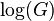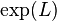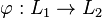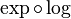# Abelian Lie correspondence

## Definition

### The setup of the correspondence

The abelian Lie correspondence, sometimes shortened as the abelian correspondence, is a special case of the Lazard correspondence, and is defined as follows:

abelian groups$\leftrightarrow$ abelian Lie rings

Here:

The abelian Lie correspondence preserves underlying sets, i.e., a group and Lie ring that are in abelian Lie correspondence have the same underlying set.

The mapping in the direction from groups to Lie rings will be denoted$\log$ and the mapping in the direction from Lie rings will be denoted$\exp$. Explicitly:

• For any abelian group$G$, we define$\log(G)$ as the Lie ring with trivial Lie bracket and with underlying additive group$G$.
• For any abelian Lie ring$L$, we define$\exp(L)$ as the underlying additive group of$L$.

### Functoriality and isomorphism of categories

Given a homomorphism of groups$\varphi: G_1 \to G_2$ of abelian groups, we can define a homomorphism of abelian Lie rings$\log\varphi: \log(G_1) \to \log(G_2)$ between their corresponding abelian Lie rings, such that both homomorphisms are the same as set maps.

Similarly, for a homomorphism$\varphi:L_1 \to L_2$ of abelian Lie rings, we can define a homomorphism$\exp(\varphi):\exp(L_1) \to \exp(L_2)$ between the corresponding abelian groups.

Thus,$\log$ and$\exp$ can be viewed as functors. Explicitly, the two categories of interest are:

• The category of abelian groups: This is the full subcategory of the category of groups whose objects are the abelian groups. Here, full subcategory means that every morphism in the bigger category between two objects in the subcategory is also in the subcategory. In this case, it means that every group homomorphism between abelian groups is a morphism in the subcategory.
• The category of abelian Lie rings: This is the full subcategory of the category of Lie rings whose objects are the abelian Lie rings.

The functors are as follows:

•$\log$ defines a functor from the category of abelian groups to the category of abelian Lie rings.
•$\exp$ defines a functor from the category of abelian Lie rings to the category of abelian groups.

The functors are two-sided inverses of each other, i.e.,$\log \circ \exp$ is the identity functor of the ctegory of abelian Lie rings and$\exp \circ \log$ is the identity functor of the category of abelian groups. Thus, the two categories are isomorphic categories.